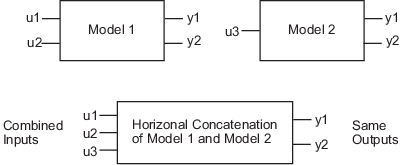Documentation

## Concatenating Models

You can perform horizontal and vertical concatenation of linear model objects to grow the number of inputs or outputs in the model.

When you concatenate identified models, such as `idtf`, `idpoly`, `idproc`, and `idss` model objects, the resulting model combines the parameters of the individual models. However, the estimated parameter covariance is lost. If you want to translate the covariance information during concatenation, use `translatecov`.

Concatenation is not supported for `idgrey` models; convert them to `idss` models first if you want to perform concatenation.

You can also concatenate nonparametric models, which contain the estimated impulse-response (`idtf` object) and frequency-response (`idfrd` object) of a system.

In case of `idfrd` models, concatenation combines information in the `ResponseData` properties of the individual model objects. `ResponseData` is an `ny`-by-`nu`-by-`nf` array that stores the response of the system, where `ny` is the number of output channels, `nu` is the number of input channels, and `nf` is the number of frequency values. The `(j,i,:)` vector of the resulting response data represents the frequency response from the `i`th input to the `j`th output at all frequencies.

### Limitation on Supported Models

Concatenation is supported for linear models only.

### Horizontal Concatenation of Model Objects

Horizontal concatenation of model objects requires that they have the same outputs. If the output channel names are different and their dimensions are the same, the concatenation operation resets the output names to their default values.

The following syntax creates a new model object `m` that contains the horizontal concatenation of `m1,m2,...,mN`:

`m = [m1,m2,...,mN]`

`m` takes all of the inputs of `m1,m2,...,mN` to the same outputs as in the original models. The following diagram is a graphical representation of horizontal concatenation of the models.### Vertical Concatenation of Model Objects

Vertical concatenation combines output channels of specified models. Vertical concatenation of model objects requires that they have the same inputs. If the input channel names are different and their dimensions are the same, the concatenation operation resets the input channel names to their default (`''`) values.

The following syntax creates a new model object `m` that contains the vertical concatenation of `m1,m2,...,mN`:

`m = [m1;m2;... ;mN]`

`m` takes the same inputs in the original models to all of the output of `m1,m2,...,mN`. The following diagram is a graphical representation of vertical concatenation of frequency-response data.### Concatenating Noise Spectrum Data of idfrd Objects

When `idfrd` models are obtained as a result of estimation (such as using `spa`), the `SpectrumData` property is not empty and contains the power spectra and cross spectra of the output noise in the system. For each output channel, this toolbox estimates one noise channel to explain the difference between the output of the model and the measured output.

When the `SpectrumData` property of individual `idfrd` objects is not empty, horizontal and vertical concatenation handle `SpectrumData`, as follows.

In case of horizontal concatenation, there is no meaningful way to combine the `SpectrumData` of individual `idfrd` objects, and the resulting `SpectrumData` property is empty. An empty property results because each `idfrd` object has its own set of noise channels, where the number of noise channels equals the number of outputs. When the resulting `idfrd` object contains the same output channels as each of the individual `idfrd` objects, it cannot accommodate the noise data from all the `idfrd` objects.

In case of vertical concatenation, this toolbox concatenates individual noise models diagonally. The following shows that `m.SpectrumData` is a block diagonal matrix of the power spectra and cross spectra of the output noise in the system:

`$m.s=\left(\begin{array}{ccc}m1.s& & 0\\ & \ddots & \\ 0& & mN.s\end{array}\right)$`

`s` in `m.s` is the abbreviation for the `SpectrumData` property name.